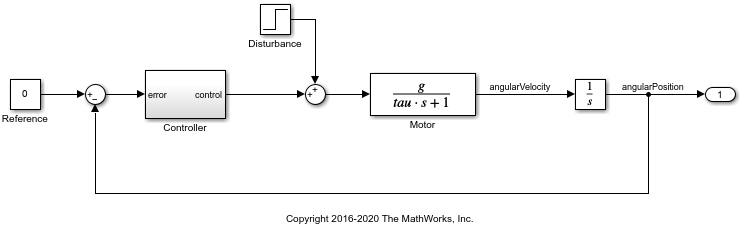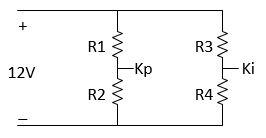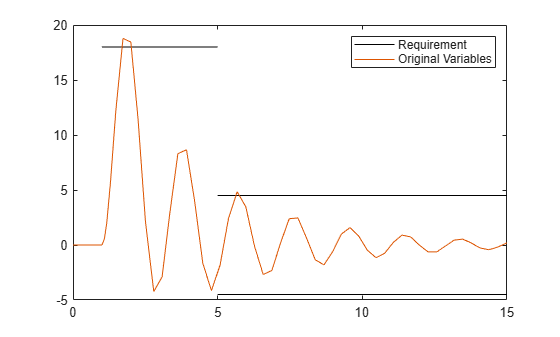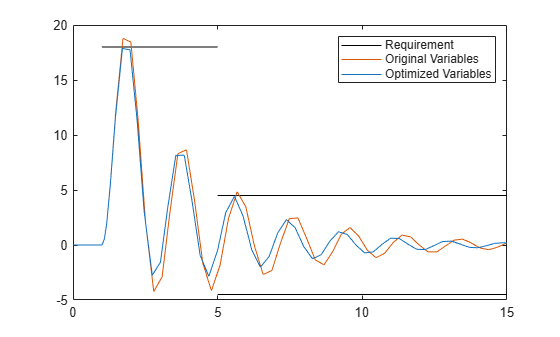# Discrete-Valued Variables in Response Optimization (Code)

This example shows how to use response optimization to tune discrete-valued variables. Discrete-valued variables represent model parameters that are restricted to a finite set of values, instead of continuously varying. To use discrete-valued variables for response optimization or parameter estimation, you must use the `surrogateopt` solver for mixed-integer optimization.

### Open the Model

In the `sdoMotorPosition` model, a PI controller enables the angular position of a DC motor to match a desired reference value. The load on the motor is subject to disturbances, and the controller must reject these disturbances.

`open_system('sdoMotorPosition')`Within the `Controller` subsystem, the PI gains are set by a 12 V source divided down by resistors `R1`, `R2`, `R3`, and `R4`, as shown in the following diagram.The proportional gain, `Kp`, is given by `12*R2/(R1+R2)` and the integral gain, `Ki`, is given by `12*R4/(R3+R4)`. The initial values of the resistances are `R1` = 47 kΩ, `R2` = 180 kΩ, `R3` = 10 kΩ, and `R4` = 10 kΩ.

### Specify Discrete Design Variables

Those four resistor values are the model parameters to tune for the optimization. Because resistors are only available in discrete values, use discrete parameters to represent them. To do so, use third input argument to `sdo.getParameterFromModel` to specify discrete parameters.

`DesignVars = sdo.getParameterFromModel('sdoMotorPosition',[],{'R1','R2','R3','R4'});`

`sdo.getParameterFromModel` returns an array of `param.Discrete` parameter objects that correspond to the model parameters in the order you specified. Set the `ValueSet` property of each parameter to the set of discrete values allowed for the parameter. Set the `Value` property to the current value, which must be present in `ValueSet`.

```% R1 values DesignVars(1).ValueSet = [39 43 47 51 56] * 1e3; DesignVars(1).Value = 47*1e3; % R2 values DesignVars(2).ValueSet = [150 160 180 200 220] * 1e3; DesignVars(2).Value = 180*1e3; % R3 values DesignVars(3).ValueSet = [8.2 9.1 10 11 12] * 1e3; DesignVars(3).Value = 10*1e3; % R4 values DesignVars(4).ValueSet = [8.2 9.1 10 11 12] * 1e3; DesignVars(4).Value = 10*1e3;```

### Specify Design Requirements and Signals

The model applies a step disturbance at 1 second. With the initial resistance values specified in the model, the disturbance causes the motor to deviate by about 20°. The response then settles back to within ±5° of the reference position by 4 seconds after the disturbance. For this example, find new resistor values to improve this specification by 10%, so that the motor deviates no more than 18°, and settles back to within ±4.5° degrees of the reference position by 4 seconds after the disturbance. First, specify these new design requirements using `sdo.requirements.SignalBound`.

```Requirements = struct; Requirements.UpperBound = sdo.requirements.SignalBound(... 'BoundMagnitudes', [18 18 ; 4.5 4.5], ... 'BoundTimes', [1 5 ; 5 15]); Requirements.LowerBound = sdo.requirements.SignalBound(... 'BoundMagnitudes', [-4.5 -4.5], ... 'BoundTimes', [5 15], ... 'Type', '>=');```

To visualize the initial performance against the requirement, first specify signals to log during simulation. In particular, log the output of the Integrator block, which is the angular position of the model, the signal you want to optimize. To do so, create a simulation scenario with `sdo.SimulationTest` and configure its `LoggingInfo` property. Also, to prepare the scenario for later use in the optimization, assign the design variables you created as its `Parameters` property.

```Simulator = sdo.SimulationTest('sdoMotorPosition'); Sig_Info = Simulink.SimulationData.SignalLoggingInfo; Sig_Info.BlockPath = 'sdoMotorPosition/Integrator'; Sig_Info.LoggingInfo.LoggingName = 'Sig'; Sig_Info.LoggingInfo.NameMode = 1; Simulator.LoggingInfo.Signals = Sig_Info; Simulator.Parameters = DesignVars;```

Now create a plot of the disturbance-rejection requirements. Then, simulate the model and add the logged signal to the plot.

```hRequirement = plot(Requirements.LowerBound.BoundTimes',Requirements.LowerBound.BoundMagnitudes','color','black'); hold on plot(Requirements.UpperBound.BoundTimes', Requirements.UpperBound.BoundMagnitudes','color','black'); % Simulator.Parameters = DesignVars; Simulator = sim(Simulator); SimLog = find(Simulator.LoggedData,get_param('sdoMotorPosition','SignalLoggingName')); Sig_Log = find(SimLog,'Sig'); clrs = colororder; hOriginal = plot(Sig_Log.Values, 'Color', clrs(2,:)); legend([hRequirement hOriginal], 'Requirement','Original Variables');```The plot shows that the current resistor values do not quite satisfy the more stringent requirement.

### Set up Optimization

The optimization process runs the model many times with different values for the resistor variables. To expedite this, configure the simulator for fast restart.

`Simulator = fastRestart(Simulator,'on');`

Optimization requires an objective or cost function that runs the model and determines whether the disturbance rejection requirements are satisfied. For this example, use the function `sdoMotorPosition_optFcn`, which is included at the end of the example. This function is called many times by the optimization solver. To pass the function to the optimizer, use an anonymous function with one argument that calls `sdoMotorPosition_optFcn`.

`optimfcn = @(P) sdoMotorPosition_optFcn(P,Simulator,Requirements);`

Specify optimization options. Set the optimization method to `surrogateopt` solver, which is the method that supports tuning of discrete parameters.

```Options = sdo.OptimizeOptions; Options.Method = 'surrogateopt'; Options.MethodOptions.MaxFunctionEvaluations = 100; Options.MethodOptions.ObjectiveLimit = 0.001; Options.OptimizedModel = Simulator;```

### Optimize the Design

To find resistance values optimized for the requirements, call `sdo.optimize` with the cost function handle, parameters to optimize, and options.

```rng('default'); % for reproducibility [Optimized_DesignVars,Info] = sdo.optimize(optimfcn,DesignVars,Options);```
``` Optimization started 26-Nov-2022 11:42:15 Current F-count max constraint max constraint Trial type 1 0.0759805 0.0759805 initial 2 0.0751287 0.0751287 random 3 0.0751287 0.179179 random 4 0.0447169 0.0447169 random 5 0.0317818 0.0317818 random 6 0.0317818 0.261353 random 7 0.0257711 0.0257711 random 8 0.0257711 0.0930365 random 9 0.0257711 0.0971938 random 10 0.0257711 0.200359 random 11 0.0062997 0.0062997 random 12 0.0062997 0.206374 random 13 0.0062997 0.0818387 random 14 0.0062997 0.0585416 random 15 0.0062997 0.114002 random 16 0.0062997 0.112485 random 17 0.0062997 0.0759805 random 18 0.0062997 0.0709489 random 19 0.0062997 0.280136 random 20 0.0062997 0.099985 random 21 -0.00737024 -0.00737024 adaptive 22 -0.00737024 0.0691728 random 23 -0.00737024 0.0347164 random 24 -0.00737024 0.0977682 random 25 -0.00737024 0.0589627 random 26 -0.00737024 0.131778 random 27 -0.00737024 0.147347 random 28 -0.00737024 0.104488 random 29 -0.00737024 0.0534262 random 30 -0.00737024 0.1643 random 31 -0.00737024 0.0419731 random 32 -0.00737024 0.0396579 random 33 -0.00737024 0.077945 random 34 -0.00737024 0.0883493 random 35 -0.00762259 -0.00762259 random 36 -0.00762259 0.0455853 random 37 -0.00762259 0.13976 random 38 -0.00762259 0.140634 random 39 -0.00762259 0.0662368 random 40 -0.00762259 0.256738 random 41 -0.00762259 0.0348764 random 42 -0.00762259 0.18995 random 43 -0.00762259 0.0615952 random 44 -0.00762259 0.0597358 random 45 -0.00762259 0.015983 random 46 -0.00762259 0.0770592 random 47 -0.00762259 0.174666 random 48 -0.00762259 0.081758 random 49 -0.00762259 0.0240828 random 50 -0.00762259 0.173292 random Current F-count max constraint max constraint Trial type 51 -0.00762259 0.116519 random 52 -0.00762259 0.217099 random 53 -0.00762259 0.104371 random 54 -0.00762259 0.0937562 random 55 -0.00762259 0.104225 random 56 -0.00762259 0.0380129 random 57 -0.00762259 0.150819 random 58 -0.00762259 0.0636666 random 59 -0.00762259 0.0320499 random 60 -0.00762259 0.0273158 random 61 -0.00762259 0.0798937 random 62 -0.00762259 0.174146 random 63 -0.00762259 0.0166127 random 64 -0.00762259 0.00433873 adaptive 65 -0.00518218 -0.00518218 adaptive 66 -0.00518218 0.294106 random 67 -0.00518218 0.0319864 random 68 -0.00518218 0.266843 random 69 -0.00518218 0.0342618 random 70 -0.00518218 0.0982987 random 71 -0.00518218 0.0419731 random 72 -0.00518218 0.0396579 random 73 -0.00518218 0.299815 random 74 -0.00518218 0.0712971 random 75 -0.00518218 0.0709489 random 76 -0.00518218 0.0589627 random 77 -0.00518218 0.131778 random 78 -0.00518218 0.00653156 random 79 -0.00518218 0.211877 random 80 -0.00518218 0.0967155 random 81 -0.00518218 0.0689487 random 82 -0.00518218 0.0257711 random 83 -0.00518218 0.0930365 random 84 -0.00518218 0.114002 random 85 -0.00518218 0.112485 random 86 -0.00518218 0.06706 random 87 -0.00495699 -0.00495699 adaptive 88 -0.00495699 0.0751287 random 89 -0.00495699 0.0759805 random 90 -0.00495699 0.10447 random 91 -0.00495699 0.190644 random 92 -0.00495699 0.142755 random 93 -0.00495699 0.104193 random 94 -0.00495699 0.100237 random 95 -0.00495699 0.097787 random 96 -0.00495699 0.0735855 random 97 -0.00495699 0.137557 random 98 -0.00495699 0.0783709 random 99 -0.00495699 0.0875674 random 100 -0.00495699 0.0794652 random 101 -0.00495699 -0.00495699 best value The current solution is feasible. surrogateopt stopped because it exceeded the function evaluation limit set by the 'MethodOptions.MaxFunctionEvaluations' property in the sdo.OptimizeOptions object. If the solution needs to be improved, you could try increasing the function evaluation limit. ```

### Evaluate Optimized Design

Plot the model response after optimization.

```Simulator.Parameters = Optimized_DesignVars; Simulator = sim(Simulator); SimLog = find(Simulator.LoggedData,get_param('sdoMotorPosition','SignalLoggingName')); Sig_Log = find(SimLog,'Sig'); clrs = colororder; hOptimized = plot(Sig_Log.Values, 'Color', clrs(1,:)); legend([hRequirement hOriginal hOptimized], 'Requirement','Original Variables','Optimized Variables');```Now the motor position is within the spec of the disturbance rejection requirement.

### Update Model with Optimized Parameter Values

`sdo.Optimize` returns tuned versions of the parameters in the array `Optimized_DesignVars`. Each entry in this array is a `param.Discrete` parameter object whose `Value` property is set to the optimized value. For instance, examine the new value of `R2`.

`Optimized_DesignVars(2).Value`
```ans = 220000 ```

Update the model with the optimized parameter values.

`sdo.setValueInModel('sdoMotorPosition',Optimized_DesignVars);`

Restore the simulator fast restart settings.

`Simulator = fastRestart(Simulator,'off');`

### Conclusion

In this example you tuned variables to improve the disturbance rejection characteristics of a motor controller. The variables being tuned were electrical component parts that could only take on discrete values, rather than continuous values. You used `sdo.getParameterFromModel` to specify the variables as discrete, and used the `surrogateopt` solver to tune the parameters.

### Objective Function

The function `sdoMotorPosition_optFcn` is called at each iteration of the optimization problem with a set of parameter values, `P`. The function returns the objective value and constraint violations, `Vals`, to the optimization solver. See `sdo.optimize` for a more detailed description of the function signature.

```function Vals = sdoMotorPosition_optFcn(P,Simulator,Requirements) %SDOMOTORPOSITION_OPTFCN % % Simulate the model. Simulator.Parameters = P; Simulator = sim(Simulator); % Retrieve logged signal data. SimLog = find(Simulator.LoggedData,get_param('sdoMotorPosition','SignalLoggingName')); Sig_Log = find(SimLog,'Sig'); % Evaluate the design requirements. Cleq_UpperBound = evalRequirement(Requirements.UpperBound,Sig_Log.Values); Cleq_LowerBound = evalRequirement(Requirements.LowerBound,Sig_Log.Values); % Collect the evaluated design requirement values in a structure to % return to the optimization solver. Vals.Cleq = [... Cleq_UpperBound(:); ... Cleq_LowerBound(:)]; end```